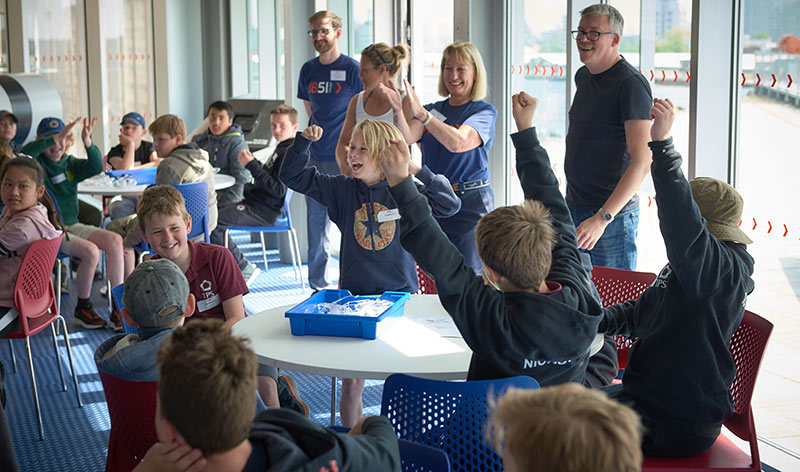# Complex Shapes and Scale Drawings

All | Maths | 11-16

## Learning Objectives

Learning about the effectiveness of renewable energy – solar panels:

1. Demonstrate how to take measurements from scale drawings.
2. Solve a problem using measurements from a scale drawing.
3. Calculate areas of complex shapes.
4. Analyse your solution to understand its impact.

## Lesson Description

The lesson is aimed at the knowledge and skills requirements for KS3 and KS4 Mathematics.

This maths topic uses the real-life context of the British America’s Cup team INEOS TEAM UK. Students will explore the modelling and data analysis behind INEOS TEAM UK’s team’s solar panel array during the 35th America’s Cup. Over two lessons, they will use the blueprint of the base’s roof to design the array, calculate area and analyse real data to evaluate the energy produced.

Use the film to discuss the motivations behind the team’s decision to install solar panels, the impact it has and the ways they monitor its efficiency and effectiveness.

The class-facing presentations provide clear lessons with differentiated worksheets to reinforce the curriculum-linked learning objectives.

This resource can be used either in sequence or flexibly as an introduction to a topic, or for a quick activity.This film introduces the use of solar panels as a renewable, sustainable way of producing electricity. Using INEOS TEAM UK's America's Cup base in Portsmouth, it explores the factors surrounding, choosing and installing solar panels. This includes the modelling required to maximise the area covered by solar panels and the data analysis that is used to understand the technology’s effectiveness and impact.

## Rating

(9)For immediate access to all of our free Key stage 3 and 4 STEM teaching resources.2.Lesson 1 Presentation Editable presentation for delivering Lesson 1 on designing a solar array using composite shapes, including Answers to the Worksheets.3.Worksheet 1-1 Explore composite shapes and calculate power output. An introduction suggested for 11-14 year olds.4.Worksheet 1-2 Explore composite shapes and calculate power output. More advanced tasks suggested for 11-14 year olds.5.Worksheet 1-3 Calculate optimum area, cost and power combinations. Suggested for 14-16 year olds.6.Base Blueprint Architectural blueprint of INEOS TEAM UK’s Base in Portsmouth required for Lesson 1.

ENGLAND
KS3 NC Maths:
Ratio, proportion and rates of change.
Model situations mathematically.
Change freely between related standard units [for example time, length, area, volume/capacity, mass].
Use scale factors, scale diagrams and maps.
Draw, measure and interpret scale drawings.
Calculate areas of composite shapes.
Understand that a multiplicative relationship between two quantities can be expressed as a ratio or a fraction.
Relate the language of ratios and the associated calculations to the arithmetic of fractions and to linear functions.
Geometry and measures.
Calculate and solve problems involving: perimeters of 2-D shapes and composite shapes.
Working mathematically.
Solve problems: begin to model situations mathematically and express the results using a range of formal mathematical representations.

KS4 NC Maths:
Compare lengths, areas and volumes using ratio notation and/or scale factors; make links to similarity.
Calculate and solve problems involving areas of composite shapes.
Convert between related compound units.
Interpret and use fractional (and negative) scale factors for enlargements.
Interpret plans.
Calculate surface areas.
GCSE Mathematics.

AQA:
R1 Change freely between related standard units.
R2 Use scale factors, scale diagrams and maps.

Edexcel:
R1 Change freely between related standard units (e.g. time, length) and compound units (e.g. speed) in numerical and algebraic contexts.
R2 use scale factors, scale diagrams and maps.
G14 use standard units of measure and related concepts (length, area, volume/capacity, mass, time, money, etc.).

OCR:
10.01c Use the scale of a map, and work with bearings. Construct and interpret scale drawings.

WALES
KS3 Maths:
Use ratio and proportion to calculate quantities, including cases where the ‘total’ is not given.

SCOTLAND
Third Level Maths – Curriculum for Excellence:
Scale drawings: word problems.
Use a formula to calculate the area of a shape.

NORTHERN IRELAND
KS3 Maths:
Recognise the need for scales and when to use them.
Identify suitable scales and scale ratios for plans and maps.
Use scales and scale ratios to read a map and calculate actual distances.
Understand terms such as plan, scale and ratio.

All, Maths

11-16

### Resource For

Students, Teachers

### Type

Base Blueprint, Film, Teacher Presentation, Worksheets

### Topic

Modelling and data analysis